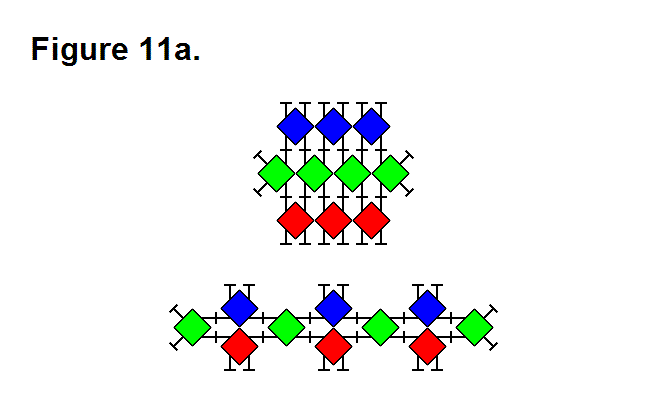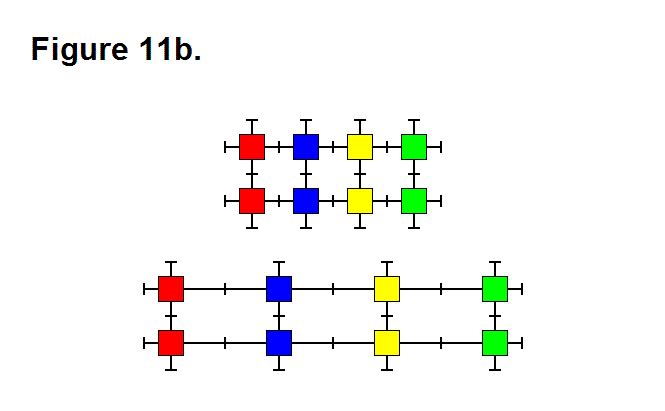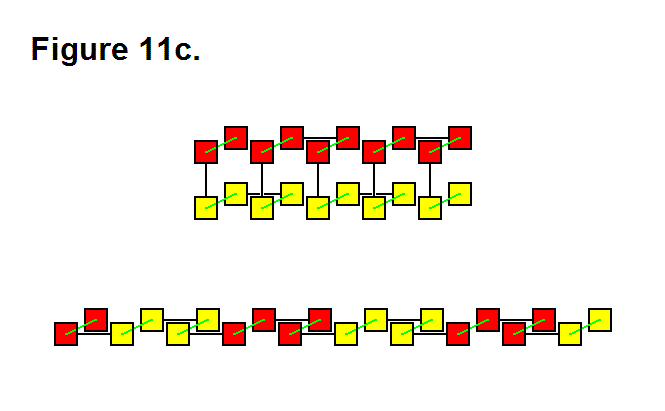# EXTENDING/RETRACTING MATRIX EFFECT

There are 3 basic means that a matrix can use to produce an extending or retracting effect; these are pivoting the legs (Figure 11a, below), telescoping the legs (Figure 11b, below), or rotating the connecting plate surface (Figure 11c, below). They can be performed in any combination with each other, and using all of them can be used to maximize the ratio between highest and lowest matrix densities. In the figures below, the upper image shows the matrix retracted and the lower image shows the matrix extended. In Figure 11c, the legs with the connecting plates that rotate are shown in green between the module cores (red and yellow rectangles) in a 2-layer 3-dimensional representation; for the upper image, the red modules are in the upper rows and the yellow modules are in the lower rows.